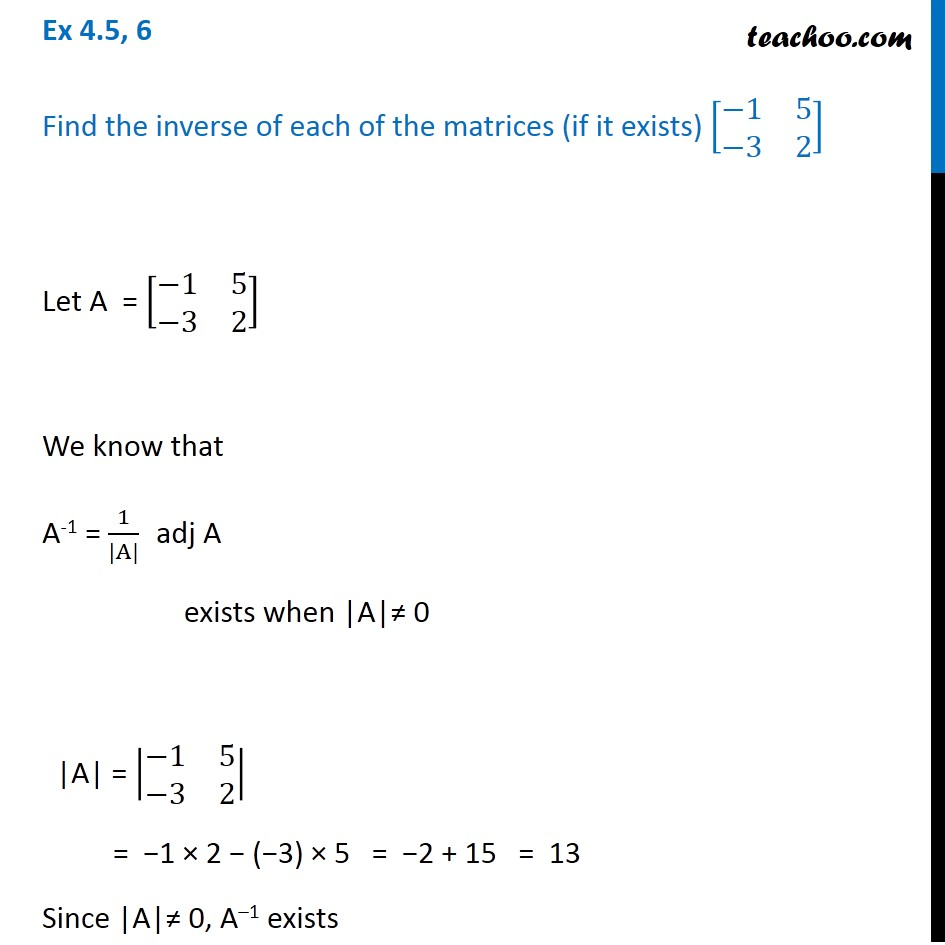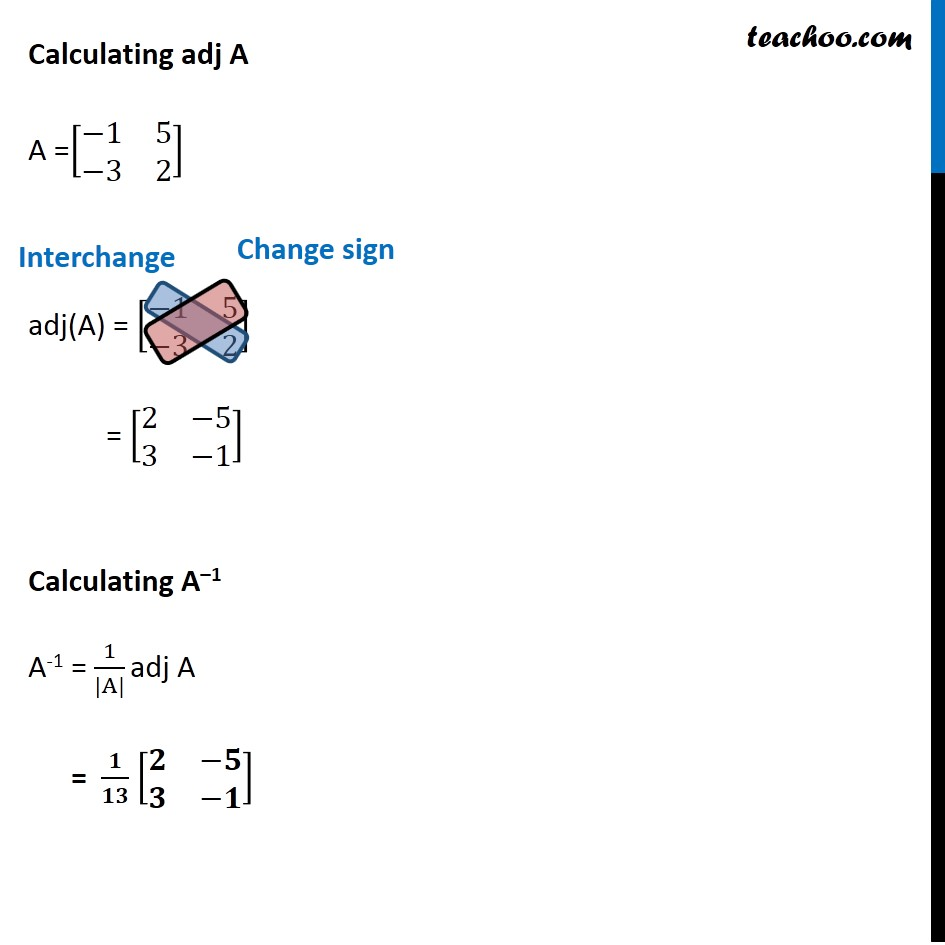Ex 4.5

Chapter 4 Class 12 Determinants (Term 1)
Serial order wise### Transcript

Ex 4.5, 6 Find the inverse of each of the matrices (if it exists) [■8(−1&5@−3&2)] Let A = [■8(−1&5@−3&2)] We know that A-1 = 1/(|A|) adj A exists when |A|≠ 0 |A| = |■8(−1&5@−3&2)| = −1 × 2 − (−3) × 5 = −2 + 15 = 13 Since |A|≠ 0, A–1 exists Calculating adj A A =[■8(−1&5@−3&2)] adj(A) = [■8(−1&5@−3&2)] = [■8(2&−5@3&−1)] Calculating A–1 A-1 = 1/(|A|) adj A = 𝟏/𝟏𝟑 [■8(𝟐&−𝟓@𝟑&−𝟏)]